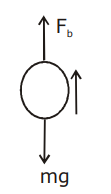# An air bubble of radius 1 cm in water has an upward acceleration 9.8 cm s–2.

An air bubble of radius 1 cm in water has an upward acceleration 9.8 cm s–2. The density of water is 1 gm cm–3 and water offers negligible drag force on the bubble. The mass of the bubble is (g = 980 cm/s2).

1) 1.52 gm

2) 4.51 gm

3) 3.15 gm

4) 4.15 gmFb–mg = ma

m=Fb/(g+ a)

m=V ρwg/(g+a)

$$m=\frac{(4/3)\pi r^{3}.\rho _{w}.g}{(g+a)}=4.15 gram$$(1)(0)